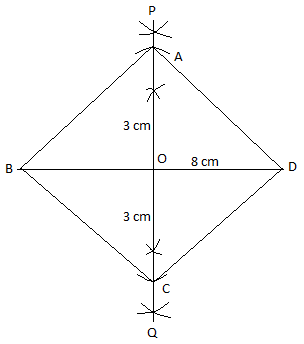Courses
Courses for Kids
Free study material
Free LIVE classes
MoreLIVE
Join Vedantu’s FREE Mastercalss

# Using ruler and compasses only, construct a rhombus whose diagonals are 8 cm and 6 cm. Measure the length of its one side.Verified
359.4k+ views
Hint: Name the rhombus and its diagonals. First draw the diagonal 8 cm. Bisect 8 cm and draw a perpendicular bisector PQ. Mark 3 cm on top and bottom of the perpendicular to get 3 cm. Join all sides and measure them.

We have to construct a rhombus when only the length of the diagonals of the rhombus is given.
Let us first name the rhombus as ABCD.
Let us consider BD as one diagonal which can be taken equal to 8 cm.
$\therefore BD=8cm$.
Let us consider the other diagonal as AC with the length of 6 cm.
$\therefore AC=6cm$.
Now, we are going to construct rhombus ABCD with diagonal lengths $BD=8cm$and $AC=6cm.$

First draw a line BD of 8 cm.
Take the bisector of BD of 8 cm. Draw the perpendicular bisector PQ of BD. Let’s take the center point of BD as ‘O’ where it is bisected.
Now mark OA = OC = 3 cm, i.e. it will be half of the diagonal of 6 cm.
Now we have to join all the sides to get the rhombus. We have drawn the two diagonals $BD=8cm$and $AC=6cm.$
Let’s join AB, AD, BC and CD.
Now if we measure side AB, we will get it as 5 cm.
Similarly, if we measure AD, BC and CD we will get it as 5cm.

To find the perpendicular bisector, take more than half of the length of side BD. Cut an arc above and below the line segment BC from point B and C. Then join the perpendicular bisector PQ.Hence, we constructed the required figure of rhombus ABCD.

Note: You can also take base as 6 cm then bisect and draw perpendicular to 6 cm. Then mark 4 cm in top and bottom of the segment. Join them to get 8 cm. Then join all the sides AB, BC, CD and DA. Still you will get the same length as 5 cm for all sides of the rhombus.
Last updated date: 22nd Sep 2023
Total views: 359.4k
Views today: 5.59k